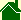#Glossary of Terms

#### Time (The Speed-of-Light Definition of Time)

A constant quantity of motion that people use to measure other motions. Through history, the motion people have called time is the apparent motion of the sun for an earthbound observer. Now, time must be redefined to be the fastest known motion -- now called the speed of light. Time is the speed of light. The specific equation for this is Time is defined equal to c.

#### The Ball-of-Light Particle Model

The Ball-of-Light Particle Model is a Grand Unification Theory. It states that all elementary particles -- that is, individual particles -- are spherical, standing waves of electric, magnetic, and gravitational fields -- that is, balls of light. The general equation for this Grand Unification Theory is Electric cross Magnetic equals Gravitational.

#### Harmonic ball-of-light

A ball-of-light that has patches of electric and magnetic fields on its surface that are: of the same magnitude, perfectly symmetrical, and non-accelerating.

#### Elementary Particle

In the Ball-of-Light Particle Model, an "elementary" particle is a "single" particle, as opposed to a collection of particles such as: atoms, molecules, people, planets, etc. The Ball-of-Light Particle Model predicts that atomic nuclei, cores of stars, and cores of galaxies are elementary particles.

#### Normal Atomic Matter

The Ball-of-Light Particle Model predicts that matter can be formed from energy. The energy range that matter can take has two extremes: neutrinos can form an elementary particle on the low end, and the entire energy of the universe can form an elementary particle on the high end. In between, energy does NOT form elementary particles in a continuous fashion. Instead, there are energy ranges were particles form harmonically, and there are energy ranges where particles form in a nonharmonic fashion. What "normal atomic matter" means in this context is simply, atoms composed from elementary particles from the lowest peak in the non-continuous spectrum of elementary particles.

Grand Unification Theory (GUT)

A Grand Unification Theory (or GUT) is a physics theory that attempts to integrate all known areas of physics into one equation or group of equations. (There have been many so-called grand unification theories in the past that have not explained time or gravity, and thus were not true grand unification theories.)

Solar Second

A "second" is a standard of motion. Visually, a second looks like the motion of a second hand on the dial face of a clock. This motion appears to be 1440 times as fast as the sun's motion across the sky appears for someone standing motionless on earth. Another way of looking at a solar second is it is exactly 1/86,400 of a day defined from when the sun is directly overhead at "true" noon.

Atomic Second

A "second" is a standard of motion. An atomic second is not based upon the sun's motion but rather on an atomic clock. This motion is almost exactly equal to a Solar Second but is minute fraction different. This difference is a result that the spin of the earth is slowing down. The Atomic Second is basically staying the same but the Solar Second is changing over time.